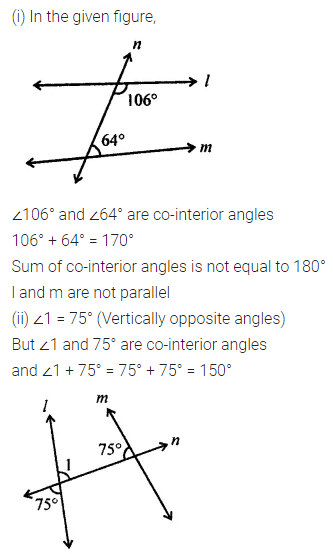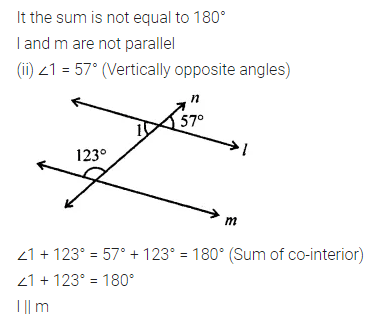# ML Aggarwal Class 7 Solutions for ICSE Maths Chapter 10 Lines and Angles Ex 10.2

## ML Aggarwal Class 7 Solutions for ICSE Maths Chapter 10 Lines and Angles Ex 10.2

Question 1.
Identify each of the given pair of angles as alternate interior angles, co-interior angles or corresponding angles or none of these in the given figure: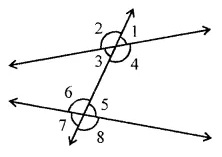(i) ∠2, ∠6
(ii) ∠1, ∠6
(iii) ∠3, ∠5
(iv) ∠2, ∠7
(v) ∠3, ∠6
(vi) ∠4, ∠8
Solution: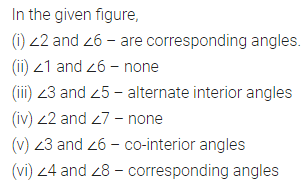Question 2.
State the property that is used in each of the following statements:
(i) If a || b, then ∠1 = ∠5.
(ii) If ∠4 = ∠6, then a || b.
(iii) If ∠4 + ∠5 = 180°, then a || b.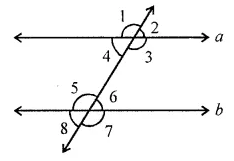Solution:Question 3.
In each of the following figures, a pair of parallel lines is cut by a transversal. Find the value of x: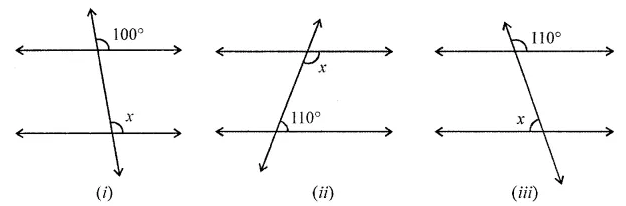Solution: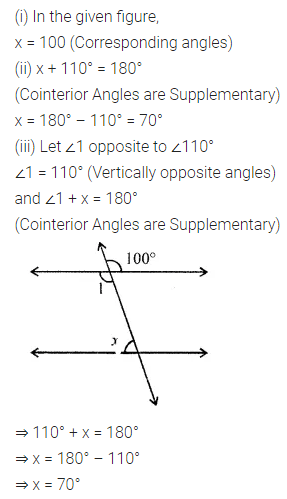Question 4.
In the following figures, a pair of parallel lines are cut by a transversal. Find the value of x in each figure.Solution: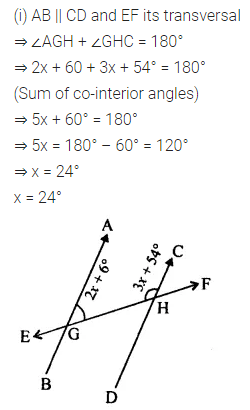Question 5.
In the following figures (i) to (vi), a pair of parallel lines are cut by a transversal. Find the size of each lettered angle.Solution: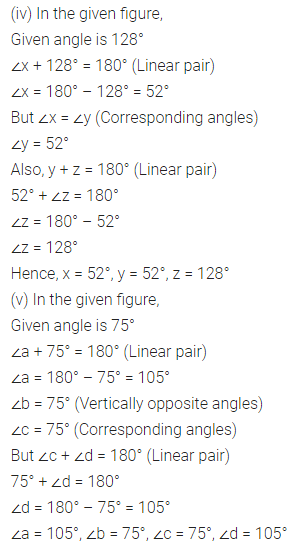Question 6.
In the given diagram, lines AB, CD and EF are parallel. Calculate the values of x and y. Hence, find the reflex angle ECA.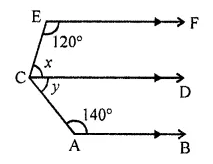Solution: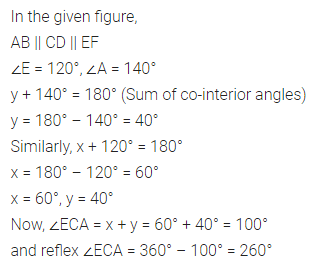Question 7.
In the given figure, l || m. Find the values of x, y and z.Solution: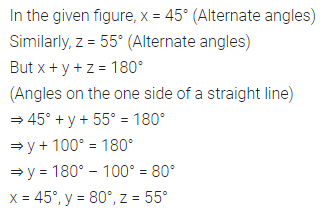Question 8.
Calculate the measure of each lettered angle in the following figure (parallel lines, segment or rays are denoted by thick matching arrows):Solution:Question 9.
In the figure given below, state whether the lines l and m are parallel or not.Solution: